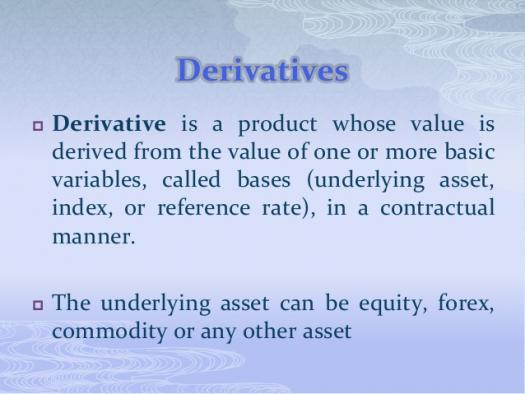# Introduction Of Derivatives Assessment Test

10 Questions | Total Attempts: 115SettingsTake our intelligent assessment test to assess your knowledge of derivatives, which is a tool of calculus, the instantaneous rate of change of a function at a point in its domain.

Related Topics
• 1.
Find the derivative of (2+3x)(1-x) with respect to x.
• A.

6x-1

• B.

1-6x

• C.

6

• D.

-3

• 2.
Find the derivative of the function y=2x2(x-1) at the point x= -1.
• A.

- 6

• B.

14

• C.

16

• D.

18

• 3.
If y= ( 1+x)2, find dy/dx.
• A.

X-1

• B.

2+2x

• C.

1+2x

• D.

2x-1

• 4.
The derivative of (2x+1) (3x+1) is...
• A.

12x+1

• B.

6x+5

• C.

6x+1

• D.

12x+1

• 5.
Find the derivative of sinb/cosb.
• A.

Sec2 b

• B.

Tan b cosec b

• C.

Cosec b sec b

• D.

Cosec2 b

• 6.
What is the derivative of t2 sin (3t - 5) with respect to the variable t?
• A.

2t sin (3t - 5) - 3t2 cos (3t - 5)

• B.

6t cos (3t - 5)

• C.

2t sin (3t - 5) + 3t2 cos (3t - 5)

• D.

2t sin (3t - 5) + t2 cos 3t

• 7.
Find the derivatives with respect to x of the function √(2 – 3×2).
• A.

-2×2 / √(2 – 3×2)

• B.

-3x / √(2 – 3×2 )

• C.

-2×2 / √(2 + 3×2)

• D.

-3x / √(2 + 3×2)

• 8.
Given the function f(x) = x to the 3rd power – 6x + 2, find the value of the first derivative at x = 2, f’(2).
• A.

6

• B.

3×2 – 5

• C.

7

• D.

9

• 9.
Find the partial derivatives with respect to x of the function: xy2 – 5y + 6.
• A.

Y2 – 5

• B.

Xy – 5y

• C.

Y2

• D.

2xy

• 10.
The derivative of ln (cos x) is...
• A.

Sec x

• B.

–sec x

• C.

–tan x

• D.

Tan x